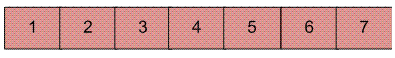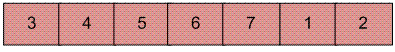# Reversal algorithm for array rotation

Write a function rotate(arr[], d, n) that rotates arr[] of size n by d elements.

Example :

```Input :  arr[] = [1, 2, 3, 4, 5, 6, 7]
d = 2
Output : arr[] = [3, 4, 5, 6, 7, 1, 2]
```Rotation of the above array by 2 will make array## Recommended: Please solve it on “PRACTICE ” first, before moving on to the solution.

The first 3 methods to rotate an array by d elements has been discussed in this post.
Method 4 (The Reversal Algorithm) :

Algorithm :

```rotate(arr[], d, n)
reverse(arr[], 1, d) ;
reverse(arr[], d + 1, n);
reverse(arr[], 1, n);
```

Let AB are the two parts of the input array where A = arr[0..d-1] and B = arr[d..n-1]. The idea of the algorithm is :

• Reverse A to get ArB, where Ar is reverse of A.
• Reverse B to get ArBr, where Br is reverse of B.
• Reverse all to get (ArBr) r = BA.

Example :
Let the array be arr[] = [1, 2, 3, 4, 5, 6, 7], d =2 and n = 7
A = [1, 2] and B = [3, 4, 5, 6, 7]

• Reverse A, we get ArB = [2, 1, 3, 4, 5, 6, 7]
• Reverse B, we get ArBr = [2, 1, 7, 6, 5, 4, 3]
• Reverse all, we get (ArBr)r = [3, 4, 5, 6, 7, 1, 2]

Below is the implementation of the above approach :

## C++

 `// C++ program for reversal algorithm ` `// of array rotation ` `#include ` `using` `namespace` `std; ` ` `  `/*Function to reverse arr[] from index start to end*/` `void` `rvereseArray(``int` `arr[], ``int` `start, ``int` `end) ` `{ ` `    ``while` `(start < end) { ` `        ``int` `temp = arr[start]; ` `        ``arr[start] = arr[end]; ` `        ``arr[end] = temp; ` `        ``start++; ` `        ``end--; ` `    ``} ` `} ` ` `  `/* Function to left rotate arr[] of size n by d */` `void` `leftRotate(``int` `arr[], ``int` `d, ``int` `n) ` `{ ` `    ``if` `(d == 0) ` `        ``return``; ` `    ``rvereseArray(arr, 0, d - 1); ` `    ``rvereseArray(arr, d, n - 1); ` `    ``rvereseArray(arr, 0, n - 1); ` `} ` ` `  `// Function to print an array ` `void` `printArray(``int` `arr[], ``int` `size) ` `{ ` `    ``for` `(``int` `i = 0; i < size; i++) ` `        ``cout << arr[i] << ``" "``; ` `} ` ` `  `/* Driver program to test above functions */` `int` `main() ` `{ ` `    ``int` `arr[] = { 1, 2, 3, 4, 5, 6, 7 }; ` `    ``int` `n = ``sizeof``(arr) / ``sizeof``(arr); ` `    ``int` `d = 2; ` ` `  `    ``// in case the rotating factor is ` `    ``// greater than array length ` `    ``d = d % n; ` ` `  `    ``// Function calling ` `    ``leftRotate(arr, d, n); ` `    ``printArray(arr, n); ` ` `  `    ``return` `0; ` `} `

## C

 `// C/C++ program for reversal algorithm of array rotation ` `#include ` ` `  `/*Utility function to print an array */` `void` `printArray(``int` `arr[], ``int` `size); ` ` `  `/* Utility function to reverse arr[] from start to end */` `void` `rvereseArray(``int` `arr[], ``int` `start, ``int` `end); ` ` `  `/* Function to left rotate arr[] of size n by d */` `void` `leftRotate(``int` `arr[], ``int` `d, ``int` `n) ` `{ ` ` `  `    ``if` `(d == 0) ` `        ``return``; ` `    ``rvereseArray(arr, 0, d - 1); ` `    ``rvereseArray(arr, d, n - 1); ` `    ``rvereseArray(arr, 0, n - 1); ` `} ` ` `  `/*UTILITY FUNCTIONS*/` `/* function to print an array */` `void` `printArray(``int` `arr[], ``int` `size) ` `{ ` `    ``int` `i; ` `    ``for` `(i = 0; i < size; i++) ` `        ``printf``(``"%d "``, arr[i]); ` `} ` ` `  `/*Function to reverse arr[] from index start to end*/` `void` `rvereseArray(``int` `arr[], ``int` `start, ``int` `end) ` `{ ` `    ``int` `temp; ` `    ``while` `(start < end) { ` `        ``temp = arr[start]; ` `        ``arr[start] = arr[end]; ` `        ``arr[end] = temp; ` `        ``start++; ` `        ``end--; ` `    ``} ` `} ` ` `  `/* Driver program to test above functions */` `int` `main() ` `{ ` `    ``int` `arr[] = { 1, 2, 3, 4, 5, 6, 7 }; ` `    ``int` `n = ``sizeof``(arr) / ``sizeof``(arr); ` `    ``int` `d = 2; ` ` `  `    ``// in case the rotating factor is ` `    ``// greater than array length ` `    ``d = d % n; ` `    ``leftRotate(arr, d, n); ` `    ``printArray(arr, n); ` `    ``return` `0; ` `} `

## Java

 `// Java program for reversal algorithm of array rotation ` `import` `java.io.*; ` ` `  `class` `LeftRotate { ` `    ``/* Function to left rotate arr[] of size n by d */` `    ``static` `void` `leftRotate(``int` `arr[], ``int` `d) ` `    ``{ ` ` `  `        ``if` `(d == ``0``) ` `            ``return``; ` `        ``int` `n = arr.length; ` `        ``rvereseArray(arr, ``0``, d - ``1``); ` `        ``rvereseArray(arr, d, n - ``1``); ` `        ``rvereseArray(arr, ``0``, n - ``1``); ` `    ``} ` ` `  `    ``/*Function to reverse arr[] from index start to end*/` `    ``static` `void` `rvereseArray(``int` `arr[], ``int` `start, ``int` `end) ` `    ``{ ` `        ``int` `temp; ` `        ``while` `(start < end) { ` `            ``temp = arr[start]; ` `            ``arr[start] = arr[end]; ` `            ``arr[end] = temp; ` `            ``start++; ` `            ``end--; ` `        ``} ` `    ``} ` ` `  `    ``/*UTILITY FUNCTIONS*/` `    ``/* function to print an array */` `    ``static` `void` `printArray(``int` `arr[]) ` `    ``{ ` `        ``for` `(``int` `i = ``0``; i < arr.length; i++) ` `            ``System.out.print(arr[i] + ``" "``); ` `    ``} ` ` `  `    ``/* Driver program to test above functions */` `    ``public` `static` `void` `main(String[] args) ` `    ``{ ` `        ``int` `arr[] = { ``1``, ``2``, ``3``, ``4``, ``5``, ``6``, ``7` `}; ` `        ``int` `n = arr.length; ` `        ``int` `d = ``2``; ` ` `  `        ``// in case the rotating factor is ` `        ``// greater than array length ` `        ``d = d % n; ` `        ``leftRotate(arr, d); ``// Rotate array by d ` `        ``printArray(arr); ` `    ``} ` `} ` `/*This code is contributed by Devesh Agrawal*/`

## Python

 `# Python program for reversal algorithm of array rotation ` ` `  `# Function to reverse arr[] from index start to end ` `def` `rverseArray(arr, start, end): ` `    ``while` `(start < end): ` `        ``temp ``=` `arr[start] ` `        ``arr[start] ``=` `arr[end] ` `        ``arr[end] ``=` `temp ` `        ``start ``+``=` `1` `        ``end ``=` `end``-``1` ` `  `# Function to left rotate arr[] of size n by d ` `def` `leftRotate(arr, d): ` ` `  `    ``if` `d ``=``=` `0``: ` `        ``return` `    ``n ``=` `len``(arr) ` `    ``rverseArray(arr, ``0``, d``-``1``) ` `    ``rverseArray(arr, d, n``-``1``) ` `    ``rverseArray(arr, ``0``, n``-``1``) ` ` `  `# Function to print an array ` `def` `printArray(arr): ` `    ``for` `i ``in` `range``(``0``, ``len``(arr)): ` `        ``print` `arr[i], ` ` `  `# Driver function to test above functions ` `arr ``=` `[``1``, ``2``, ``3``, ``4``, ``5``, ``6``, ``7``] ` `n ``=` `len``(arr) ` `d ``=` `2` ` `  `# in case the rotating factor is  ` `# greater than array length ` `d ``=` `d ``%` `n       ` `leftRotate(arr, d) ``# Rotate array by 2 ` `printArray(arr) ` ` `  `# This code is contributed by Devesh Agrawal `

## C#

 `// C# program for reversal algorithm ` `// of array rotation ` `using` `System; ` ` `  `class` `GFG { ` `    ``/* Function to left rotate arr[] ` `    ``of size n by d */` `    ``static` `void` `leftRotate(``int``[] arr, ``int` `d) ` `    ``{ ` ` `  `        ``if` `(d == 0) ` `            ``return``; ` `        ``int` `n = arr.Length; ` `        ``rvereseArray(arr, 0, d - 1); ` `        ``rvereseArray(arr, d, n - 1); ` `        ``rvereseArray(arr, 0, n - 1); ` `    ``} ` ` `  `    ``/* Function to reverse arr[] from ` `    ``index start to end*/` `    ``static` `void` `rvereseArray(``int``[] arr, ``int` `start, ` `                             ``int` `end) ` `    ``{ ` `        ``int` `temp; ` `        ``while` `(start < end) { ` `            ``temp = arr[start]; ` `            ``arr[start] = arr[end]; ` `            ``arr[end] = temp; ` `            ``start++; ` `            ``end--; ` `        ``} ` `    ``} ` ` `  `    ``/*UTILITY FUNCTIONS*/` `    ``/* function to print an array */` `    ``static` `void` `printArray(``int``[] arr) ` `    ``{ ` `        ``for` `(``int` `i = 0; i < arr.Length; i++) ` `            ``Console.Write(arr[i] + ``" "``); ` `    ``} ` ` `  `    ``// Driver code ` `    ``public` `static` `void` `Main() ` `    ``{ ` `        ``int``[] arr = { 1, 2, 3, 4, 5, 6, 7 }; ` `        ``int` `n = arr.Length; ` `        ``int` `d = 2; ` ` `  `        ``// in case the rotating factor is ` `        ``// greater than array length ` `        ``d = d % n; ` `        ``leftRotate(arr, 2); ``// Rotate array by 2 ` `        ``printArray(arr); ` `    ``} ` `} ` ` `  `// This code is contributed by Sam007 `

## PHP

 ` `

Output :

`3 4 5 6 7 1 2`

Time Complexity : O(n)

Don’t stop now and take your learning to the next level. Learn all the important concepts of Data Structures and Algorithms with the help of the most trusted course: DSA Self Paced. Become industry ready at a student-friendly price.

My Personal Notes arrow_drop_up

Article Tags :
Practice Tags :

160

Please write to us at contribute@geeksforgeeks.org to report any issue with the above content.